# Recurrent Neural Network with PyTorch¶

Run Jupyter Notebook

You can run the code for this section in this jupyter notebook link.

### Feedforward Neural Networks Transition to 1 Layer Recurrent Neural Networks (RNN)¶

• RNN is essentially an FNN but with a hidden layer (non-linear output) that passes on information to the next FNN
• Compared to an FNN, we've one additional set of weight and bias that allows information to flow from one FNN to another FNN sequentially that allows time-dependency.
• The diagram below shows the only difference between an FNN and a RNN.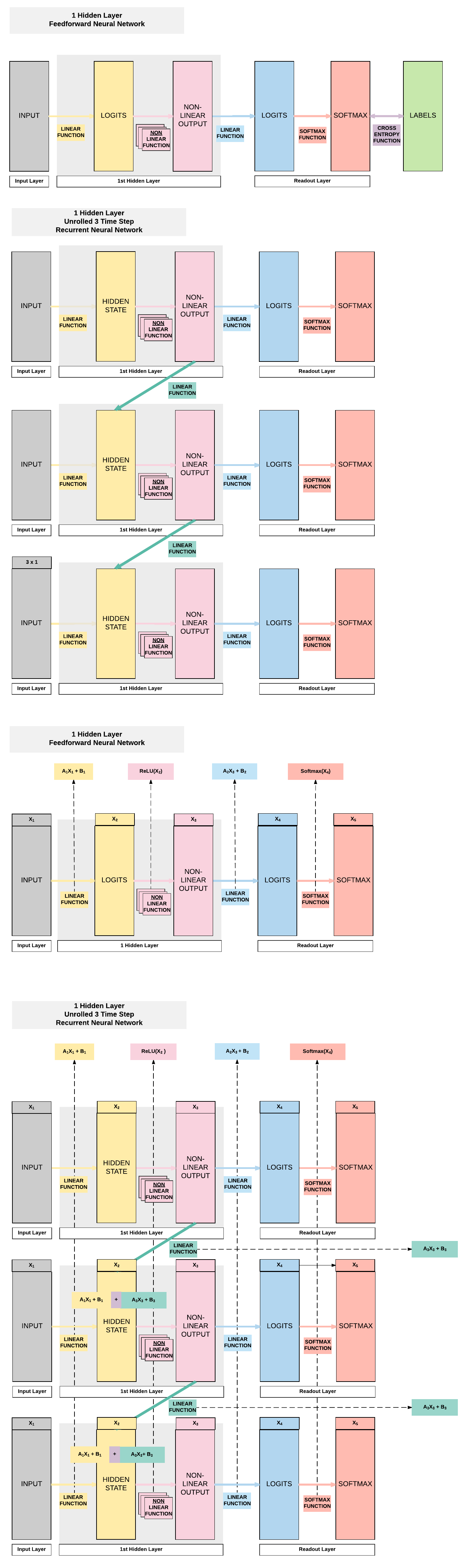### 2 Layer RNN Breakdown¶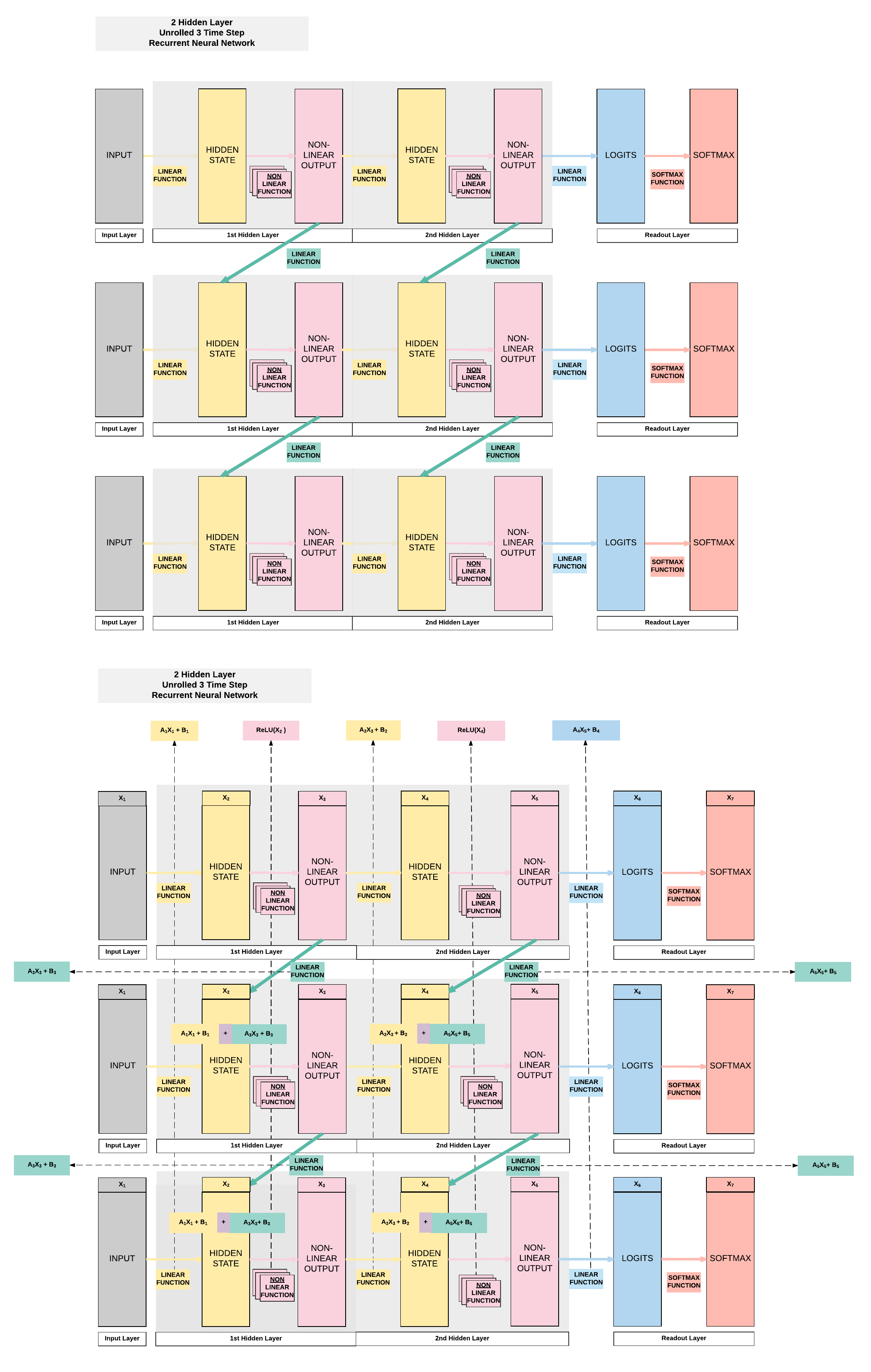## Building a Recurrent Neural Network with PyTorch¶

### Model A: 1 Hidden Layer (ReLU)¶

• Unroll 28 time steps
• Each step input size: 28 x 1
• Total per unroll: 28 x 28
• Feedforward Neural Network input size: 28 x 28
• 1 Hidden layer
• ReLU Activation Function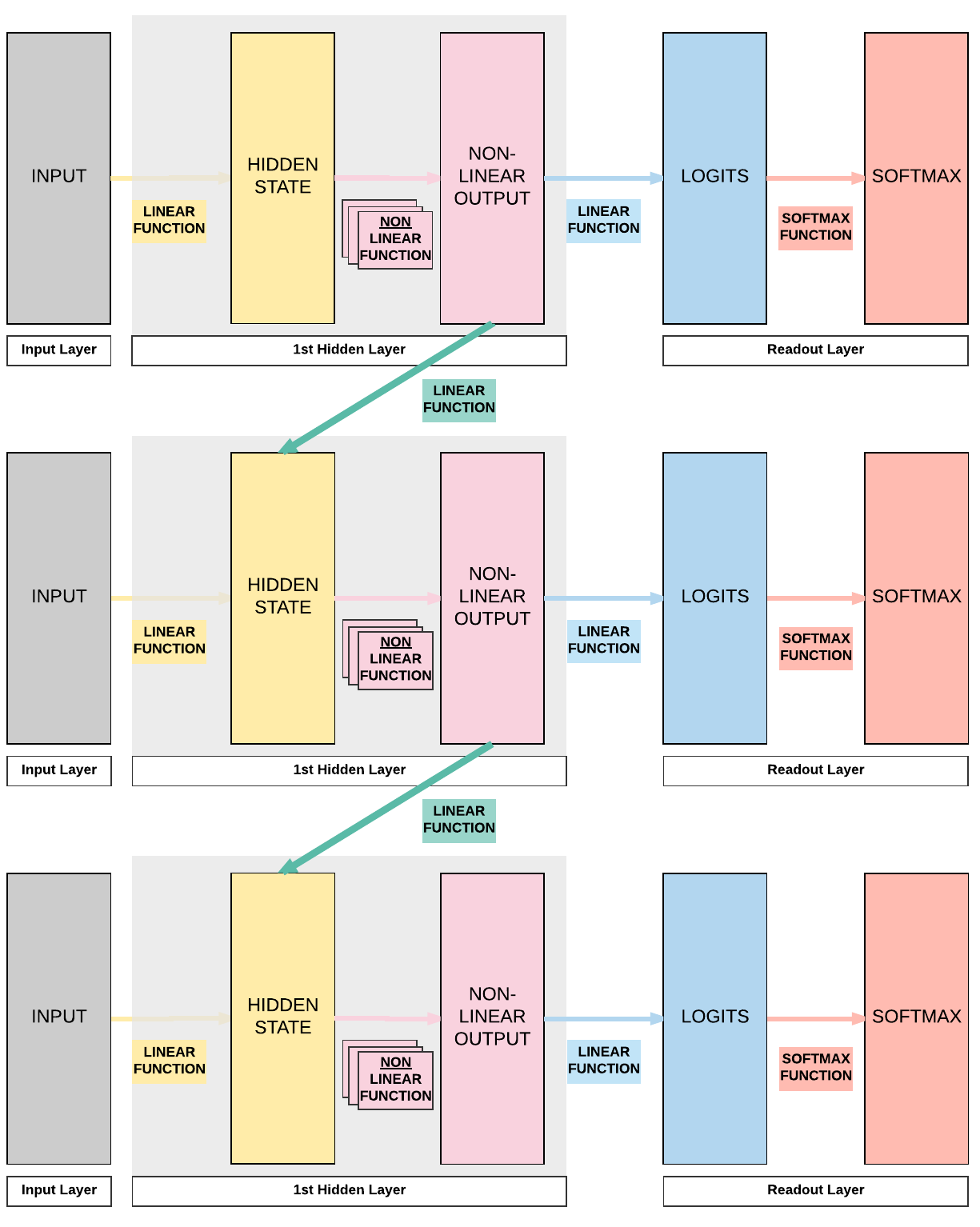#### Steps¶

• Step 2: Make Dataset Iterable
• Step 3: Create Model Class
• Step 4: Instantiate Model Class
• Step 5: Instantiate Loss Class
• Step 6: Instantiate Optimizer Class
• Step 7: Train Model

Images from 1 to 9

Looking into the MNIST Dataset

import torch
import torch.nn as nn
import torchvision.transforms as transforms
import torchvision.datasets as dsets

train_dataset = dsets.MNIST(root='./data',
train=True,
transform=transforms.ToTensor(),

test_dataset = dsets.MNIST(root='./data',
train=False,
transform=transforms.ToTensor())


We would have 60k training images of size 28 x 28 pixels.

print(train_dataset.train_data.size())

print(train_dataset.train_labels.size())


Here we would have 10k testing images of the same size, 28 x 28 pixels.

print(test_dataset.test_data.size())

print(test_dataset.test_labels.size())

torch.Size([60000, 28, 28])

torch.Size()

torch.Size([10000, 28, 28])

torch.Size()


#### Step 2: Make Dataset Iterable¶

Creating iterable objects to loop through subsequently

batch_size = 100
n_iters = 3000
num_epochs = n_iters / (len(train_dataset) / batch_size)
num_epochs = int(num_epochs)

batch_size=batch_size,
shuffle=True)

batch_size=batch_size,
shuffle=False)


#### Step 3: Create Model Class¶

1 Layer RNNclass RNNModel(nn.Module):
def __init__(self, input_dim, hidden_dim, layer_dim, output_dim):
super(RNNModel, self).__init__()
# Hidden dimensions
self.hidden_dim = hidden_dim

# Number of hidden layers
self.layer_dim = layer_dim

# batch_first=True causes input/output tensors to be of shape
# (batch_dim, seq_dim, input_dim)
# batch_dim = number of samples per batch
self.rnn = nn.RNN(input_dim, hidden_dim, layer_dim, batch_first=True, nonlinearity='relu')

self.fc = nn.Linear(hidden_dim, output_dim)

def forward(self, x):
# Initialize hidden state with zeros
# (layer_dim, batch_size, hidden_dim)

# We need to detach the hidden state to prevent exploding/vanishing gradients
# This is part of truncated backpropagation through time (BPTT)
out, hn = self.rnn(x, h0.detach())

# Index hidden state of last time step
# out.size() --> 100, 28, 10
# out[:, -1, :] --> 100, 10 --> just want last time step hidden states!
out = self.fc(out[:, -1, :])
# out.size() --> 100, 10
return out


#### Step 4: Instantiate Model Class¶

• 28 time steps
• Each time step: input dimension = 28
• 1 hidden layer
• MNIST 1-9 digits $\rightarrow$ output dimension = 10

Instantiate model class and assign to an object

input_dim = 28
hidden_dim = 100
layer_dim = 1
output_dim = 10

model = RNNModel(input_dim, hidden_dim, layer_dim, output_dim)


#### Step 5: Instantiate Loss Class¶

• Recurrent Neural Network: Cross Entropy Loss
• Convolutional Neural Network: Cross Entropy Loss
• Feedforward Neural Network: Cross Entropy Loss
• Logistic Regression: Cross Entropy Loss
• Linear Regression: MSE

Cross Entropy Loss for Classification Task

criterion = nn.CrossEntropyLoss()


Cross Entropy vs MSE

Take note that there are cases where RNN, CNN and FNN use MSE as a loss function.

We use cross entropy for classification tasks (predicting 0-9 digits in MNIST for example).

And we use MSE for regression tasks (predicting temperatures in every December in San Francisco for example).

#### Step 6: Instantiate Optimizer Class¶

• Simplified equation
• $\theta = \theta - \eta \cdot \nabla_\theta$
• $\theta$: parameters (our tensors with gradient accumulation abilities)
• $\eta$: learning rate (how fast we want to learn)
• $\nabla_\theta$: gradients of loss with respect to the model's parameters
• Even simplier equation
• parameters = parameters - learning_rate * parameters_gradients
• At every iteration, we update our model's parameters
learning_rate = 0.01

optimizer = torch.optim.SGD(model.parameters(), lr=learning_rate)

###### Parameters In-Depth¶
• Input to Hidden Layer Affine Function
• A1, B1
• Hidden Layer to Output Affine Function
• A2, B2
• Hidden Layer to Hidden Layer Affine Function
• A3, B3Total groups of parameters

We should have 6 groups as shown above.

len(list(model.parameters()))

6


Input to Hidden Weight

Remember we defined our hidden layer to have a size of 100. Because our input is a size of 28 at each time step, this gives rise to a weight matrix of 100 x 28.

# Input --> Hidden (A1)
list(model.parameters()).size()

torch.Size([100, 28])


Input to Hidden Bias

# Input --> Hidden BIAS (B1)
list(model.parameters()).size()

torch.Size()


Hidden to Hidden

# Hidden --> Hidden (A3)
list(model.parameters()).size()

torch.Size([100, 100])


Hidden to Hidden Bias

# Hidden --> Hidden BIAS(B3)
list(model.parameters()).size()

torch.Size()


Hidden to Output

# Hidden --> Output (A2)
list(model.parameters()).size()

torch.Size([10, 100])


Hidden to Output Bias

# Hidden --> Output BIAS (B2)
list(model.parameters()).size()

torch.Size()


#### Step 7: Train Model¶

• Process
1. Convert inputs/labels to tensors with gradient accumulation abilities
• RNN Input: (1, 28)
• CNN Input: (1, 28, 28)
• FNN Input: (1, 28*28)
3. Get output given inputs
4. Get loss
• parameters = parameters - learning_rate * parameters_gradients
7. REPEAT

Same 7 step process for training models

# Number of steps to unroll
seq_dim = 28

iter = 0
for epoch in range(num_epochs):
for i, (images, labels) in enumerate(train_loader):
model.train()

# Forward pass to get output/logits
# outputs.size() --> 100, 10
outputs = model(images)

# Calculate Loss: softmax --> cross entropy loss
loss = criterion(outputs, labels)

loss.backward()

# Updating parameters
optimizer.step()

iter += 1

if iter % 500 == 0:
model.eval()
# Calculate Accuracy
correct = 0
total = 0
# Iterate through test dataset
images = images.view(-1, seq_dim, input_dim)

# Forward pass only to get logits/output
outputs = model(images)

# Get predictions from the maximum value
_, predicted = torch.max(outputs.data, 1)

# Total number of labels
total += labels.size(0)

# Total correct predictions
correct += (predicted == labels).sum()

accuracy = 100 * correct / total

# Print Loss
print('Iteration: {}. Loss: {}. Accuracy: {}'.format(iter, loss.item(), accuracy))

Iteration: 500. Loss: 2.301494836807251. Accuracy: 12
Iteration: 1000. Loss: 2.2986037731170654. Accuracy: 14
Iteration: 1500. Loss: 2.278566598892212. Accuracy: 18
Iteration: 2000. Loss: 2.169614315032959. Accuracy: 21
Iteration: 2500. Loss: 1.1662731170654297. Accuracy: 51
Iteration: 3000. Loss: 0.9290509223937988. Accuracy: 71


### Model B: 2 Hidden Layer (ReLU)¶

• Unroll 28 time steps
• Each step input size: 28 x 1
• Total per unroll: 28 x 28
• Feedforward Neural Network inpt size: 28 x 28
• 2 Hidden layer
• ReLU Activation Function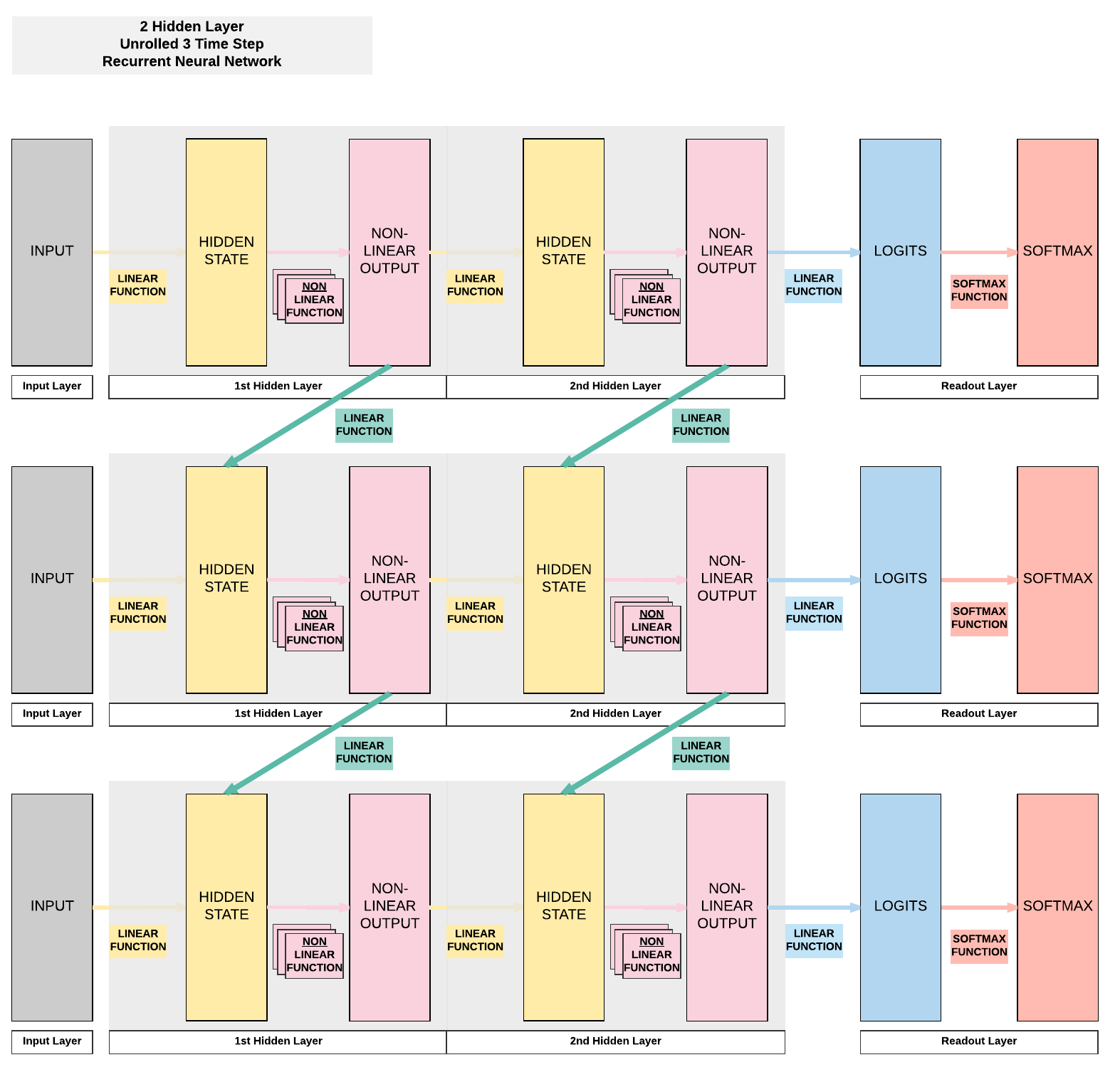#### Steps¶

• Step 2: Make Dataset Iterable
• Step 3: Create Model Class
• Step 4: Instantiate Model Class
• Step 5: Instantiate Loss Class
• Step 6: Instantiate Optimizer Class
• Step 7: Train Model

2 Hidden Layer + ReLU

import torch
import torch.nn as nn
import torchvision.transforms as transforms
import torchvision.datasets as dsets

'''
'''
train_dataset = dsets.MNIST(root='./data',
train=True,
transform=transforms.ToTensor(),

test_dataset = dsets.MNIST(root='./data',
train=False,
transform=transforms.ToTensor())

'''
STEP 2: MAKING DATASET ITERABLE
'''

batch_size = 100
n_iters = 3000
num_epochs = n_iters / (len(train_dataset) / batch_size)
num_epochs = int(num_epochs)

batch_size=batch_size,
shuffle=True)

batch_size=batch_size,
shuffle=False)

'''
STEP 3: CREATE MODEL CLASS
'''

class RNNModel(nn.Module):
def __init__(self, input_dim, hidden_dim, layer_dim, output_dim):
super(RNNModel, self).__init__()
# Hidden dimensions
self.hidden_dim = hidden_dim

# Number of hidden layers
self.layer_dim = layer_dim

# batch_first=True causes input/output tensors to be of shape
# (batch_dim, seq_dim, feature_dim)
self.rnn = nn.RNN(input_dim, hidden_dim, layer_dim, batch_first=True, nonlinearity='relu')

self.fc = nn.Linear(hidden_dim, output_dim)

def forward(self, x):
# Initialize hidden state with zeros

# We need to detach the hidden state to prevent exploding/vanishing gradients
# This is part of truncated backpropagation through time (BPTT)
out, hn = self.rnn(x, h0.detach())

# Index hidden state of last time step
# out.size() --> 100, 28, 100
# out[:, -1, :] --> 100, 100 --> just want last time step hidden states!
out = self.fc(out[:, -1, :])
# out.size() --> 100, 10
return out

'''
STEP 4: INSTANTIATE MODEL CLASS
'''
input_dim = 28
hidden_dim = 100
layer_dim = 2  # ONLY CHANGE IS HERE FROM ONE LAYER TO TWO LAYER
output_dim = 10

model = RNNModel(input_dim, hidden_dim, layer_dim, output_dim)

# JUST PRINTING MODEL & PARAMETERS
print(model)
print(len(list(model.parameters())))
for i in range(len(list(model.parameters()))):
print(list(model.parameters())[i].size())

'''
STEP 5: INSTANTIATE LOSS CLASS
'''
criterion = nn.CrossEntropyLoss()

'''
STEP 6: INSTANTIATE OPTIMIZER CLASS
'''
learning_rate = 0.01

optimizer = torch.optim.SGD(model.parameters(), lr=learning_rate)

'''
STEP 7: TRAIN THE MODEL
'''

# Number of steps to unroll
seq_dim = 28

iter = 0
for epoch in range(num_epochs):
for i, (images, labels) in enumerate(train_loader):
model.train()

# Forward pass to get output/logits
# outputs.size() --> 100, 10
outputs = model(images)

# Calculate Loss: softmax --> cross entropy loss
loss = criterion(outputs, labels)

loss.backward()

# Updating parameters
optimizer.step()

iter += 1

if iter % 500 == 0:
model.eval()
# Calculate Accuracy
correct = 0
total = 0
# Iterate through test dataset
# Resize images
images = images.view(-1, seq_dim, input_dim)

# Forward pass only to get logits/output
outputs = model(images)

# Get predictions from the maximum value
_, predicted = torch.max(outputs.data, 1)

# Total number of labels
total += labels.size(0)

# Total correct predictions
correct += (predicted == labels).sum()

accuracy = 100 * correct / total

# Print Loss
print('Iteration: {}. Loss: {}. Accuracy: {}'.format(iter, loss.item(), accuracy))

RNNModel(
(rnn): RNN(28, 100, num_layers=2, batch_first=True)
(fc): Linear(in_features=100, out_features=10, bias=True)
)

10

torch.Size([100, 28])
torch.Size([100, 100])
torch.Size()
torch.Size()
torch.Size([100, 100])
torch.Size([100, 100])
torch.Size()
torch.Size()
torch.Size([10, 100])
torch.Size()

Iteration: 500. Loss: 2.3019518852233887. Accuracy: 11
Iteration: 1000. Loss: 2.299217700958252. Accuracy: 11
Iteration: 1500. Loss: 2.279090166091919. Accuracy: 14
Iteration: 2000. Loss: 2.126953125. Accuracy: 25
Iteration: 2500. Loss: 1.356347680091858. Accuracy: 57
Iteration: 3000. Loss: 0.7377720475196838. Accuracy: 69

• 10 sets of parameters
• First hidden Layer
• $A_1 = [100, 28]$
• $A_3 = [100, 100]$
• $B_1 = $
• $B_3 = $
• Second hidden layer
• $A_2 = [100, 100]$
• $A_5 = [100, 100]$
• $B_2 = $
• $B_5 = $
• $A_4 = [10, 100]$
• $B_4 = $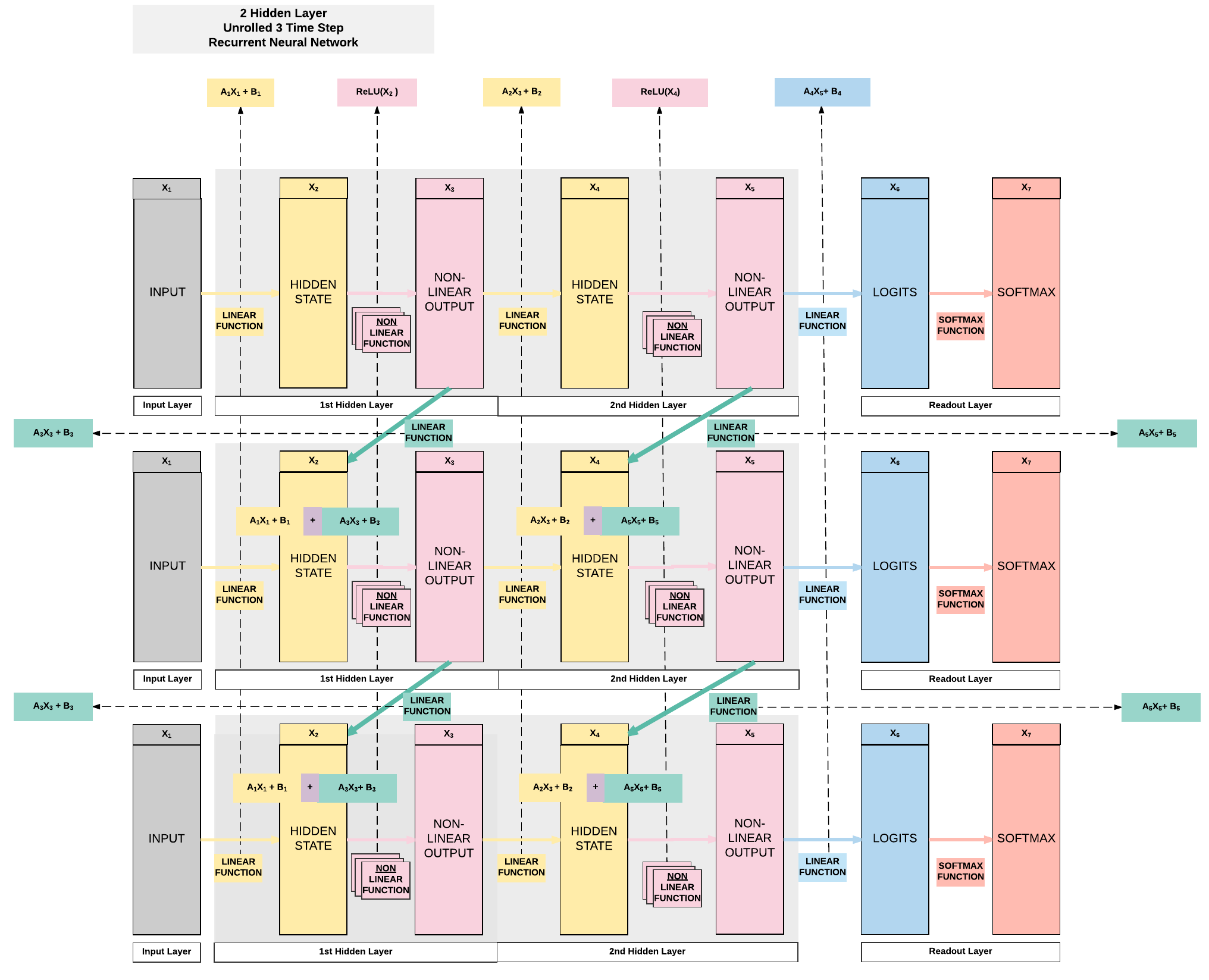### Model C: 2 Hidden Layer¶

• Unroll 28 time steps
• Each step input size: 28 x 1
• Total per unroll: 28 x 28
• Feedforward Neural Network inpt size: 28 x 28
• 2 Hidden layer
• Tanh Activation Function#### Steps¶

• Step 2: Make Dataset Iterable
• Step 3: Create Model Class
• Step 4: Instantiate Model Class
• Step 5: Instantiate Loss Class
• Step 6: Instantiate Optimizer Class
• Step 7: Train Model

!!! "2 Hidden + ReLU"

import torch
import torch.nn as nn
import torchvision.transforms as transforms
import torchvision.datasets as dsets

'''
'''
train_dataset = dsets.MNIST(root='./data',
train=True,
transform=transforms.ToTensor(),

test_dataset = dsets.MNIST(root='./data',
train=False,
transform=transforms.ToTensor())

'''
STEP 2: MAKING DATASET ITERABLE
'''

batch_size = 100
n_iters = 3000
num_epochs = n_iters / (len(train_dataset) / batch_size)
num_epochs = int(num_epochs)

batch_size=batch_size,
shuffle=True)

batch_size=batch_size,
shuffle=False)

'''
STEP 3: CREATE MODEL CLASS
'''

class RNNModel(nn.Module):
def __init__(self, input_dim, hidden_dim, layer_dim, output_dim):
super(RNNModel, self).__init__()
# Hidden dimensions
self.hidden_dim = hidden_dim

# Number of hidden layers
self.layer_dim = layer_dim

# batch_first=True causes input/output tensors to be of shape
# (batch_dim, seq_dim, feature_dim)
self.rnn = nn.RNN(input_dim, hidden_dim, layer_dim, batch_first=True, nonlinearity='tanh')

self.fc = nn.Linear(hidden_dim, output_dim)

def forward(self, x):
# Initialize hidden state with zeros

# One time step
# We need to detach the hidden state to prevent exploding/vanishing gradients
# This is part of truncated backpropagation through time (BPTT)
out, hn = self.rnn(x, h0.detach())

# Index hidden state of last time step
# out.size() --> 100, 28, 100
# out[:, -1, :] --> 100, 100 --> just want last time step hidden states!
out = self.fc(out[:, -1, :])
# out.size() --> 100, 10
return out

'''
STEP 4: INSTANTIATE MODEL CLASS
'''
input_dim = 28
hidden_dim = 100
layer_dim = 2  # ONLY CHANGE IS HERE FROM ONE LAYER TO TWO LAYER
output_dim = 10

model = RNNModel(input_dim, hidden_dim, layer_dim, output_dim)

# JUST PRINTING MODEL & PARAMETERS
print(model)
print(len(list(model.parameters())))
for i in range(len(list(model.parameters()))):
print(list(model.parameters())[i].size())

'''
STEP 5: INSTANTIATE LOSS CLASS
'''
criterion = nn.CrossEntropyLoss()

'''
STEP 6: INSTANTIATE OPTIMIZER CLASS
'''
learning_rate = 0.1

optimizer = torch.optim.SGD(model.parameters(), lr=learning_rate)

'''
STEP 7: TRAIN THE MODEL
'''

# Number of steps to unroll
seq_dim = 28

iter = 0
for epoch in range(num_epochs):
for i, (images, labels) in enumerate(train_loader):

# Forward pass to get output/logits
# outputs.size() --> 100, 10
outputs = model(images)

# Calculate Loss: softmax --> cross entropy loss
loss = criterion(outputs, labels)

loss.backward()

# Updating parameters
optimizer.step()

iter += 1

if iter % 500 == 0:
# Calculate Accuracy
correct = 0
total = 0
# Iterate through test dataset
# Resize images
images = images.view(-1, seq_dim, input_dim)

# Forward pass only to get logits/output
outputs = model(images)

# Get predictions from the maximum value
_, predicted = torch.max(outputs.data, 1)

# Total number of labels
total += labels.size(0)

# Total correct predictions
correct += (predicted == labels).sum()

accuracy = 100 * correct / total

# Print Loss
print('Iteration: {}. Loss: {}. Accuracy: {}'.format(iter, loss.item(), accuracy))


RNNModel(
(rnn): RNN(28, 100, num_layers=2, batch_first=True)
(fc): Linear(in_features=100, out_features=10, bias=True)
)

10

torch.Size([100, 28])
torch.Size([100, 100])
torch.Size()
torch.Size()
torch.Size([100, 100])
torch.Size([100, 100])
torch.Size()
torch.Size()
torch.Size([10, 100])
torch.Size()
Iteration: 500. Loss: 0.5943437218666077. Accuracy: 77
Iteration: 1000. Loss: 0.22048641741275787. Accuracy: 91
Iteration: 1500. Loss: 0.18479223549365997. Accuracy: 94
Iteration: 2000. Loss: 0.2723771929740906. Accuracy: 91
Iteration: 2500. Loss: 0.18817797303199768. Accuracy: 92
Iteration: 3000. Loss: 0.1685929149389267. Accuracy: 92


## Summary of Results¶

Model A Model B Model C
ReLU ReLU Tanh
1 Hidden Layer 2 Hidden Layers 2 Hidden Layers
100 Hidden Units 100 Hidden Units 100 Hidden Units
92.48% 95.09% 95.54%

## General Deep Learning Notes¶

• 2 ways to expand a recurrent neural network
• More non-linear activation units (neurons)
• More hidden layers
• Cons
• Need a larger dataset
• Curse of dimensionality
• Does not necessarily mean higher accuracy

## 3. Building a Recurrent Neural Network with PyTorch (GPU)¶

### Model C: 2 Hidden Layer (Tanh)¶GPU: 2 things must be on GPU - model - tensors

### Steps¶

• Step 2: Make Dataset Iterable
• Step 3: Create Model Class
• Step 4: Instantiate Model Class
• Step 5: Instantiate Loss Class
• Step 6: Instantiate Optimizer Class
• Step 7: Train Model

2 Layer RNN + Tanh

import torch
import torch.nn as nn
import torchvision.transforms as transforms
import torchvision.datasets as dsets

'''
'''
train_dataset = dsets.MNIST(root='./data',
train=True,
transform=transforms.ToTensor(),

test_dataset = dsets.MNIST(root='./data',
train=False,
transform=transforms.ToTensor())

'''
STEP 2: MAKING DATASET ITERABLE
'''

batch_size = 100
n_iters = 3000
num_epochs = n_iters / (len(train_dataset) / batch_size)
num_epochs = int(num_epochs)

batch_size=batch_size,
shuffle=True)

batch_size=batch_size,
shuffle=False)

'''
STEP 3: CREATE MODEL CLASS
'''

class RNNModel(nn.Module):
def __init__(self, input_dim, hidden_dim, layer_dim, output_dim):
super(RNNModel, self).__init__()
# Hidden dimensions
self.hidden_dim = hidden_dim

# Number of hidden layers
self.layer_dim = layer_dim

# batch_first=True causes input/output tensors to be of shape
# (batch_dim, seq_dim, feature_dim)
self.rnn = nn.RNN(input_dim, hidden_dim, layer_dim, batch_first=True, nonlinearity='tanh')

self.fc = nn.Linear(hidden_dim, output_dim)

def forward(self, x):
# Initialize hidden state with zeros
#######################
#  USE GPU FOR MODEL  #
#######################
h0 = torch.zeros(self.layer_dim, x.size(0), self.hidden_dim).to(device)

# One time step
# We need to detach the hidden state to prevent exploding/vanishing gradients
# This is part of truncated backpropagation through time (BPTT)
out, hn = self.rnn(x, h0.detach())

# Index hidden state of last time step
# out.size() --> 100, 28, 100
# out[:, -1, :] --> 100, 100 --> just want last time step hidden states!
out = self.fc(out[:, -1, :])
# out.size() --> 100, 10
return out

'''
STEP 4: INSTANTIATE MODEL CLASS
'''
input_dim = 28
hidden_dim = 100
layer_dim = 2  # ONLY CHANGE IS HERE FROM ONE LAYER TO TWO LAYER
output_dim = 10

model = RNNModel(input_dim, hidden_dim, layer_dim, output_dim)

#######################
#  USE GPU FOR MODEL  #
#######################

device = torch.device("cuda:0" if torch.cuda.is_available() else "cpu")
model.to(device)

'''
STEP 5: INSTANTIATE LOSS CLASS
'''
criterion = nn.CrossEntropyLoss()

'''
STEP 6: INSTANTIATE OPTIMIZER CLASS
'''
learning_rate = 0.1

optimizer = torch.optim.SGD(model.parameters(), lr=learning_rate)

'''
STEP 7: TRAIN THE MODEL
'''

# Number of steps to unroll
seq_dim = 28

iter = 0
for epoch in range(num_epochs):
for i, (images, labels) in enumerate(train_loader):
#######################
#  USE GPU FOR MODEL  #
#######################
labels = labels.to(device)

# Forward pass to get output/logits
# outputs.size() --> 100, 10
outputs = model(images)

# Calculate Loss: softmax --> cross entropy loss
loss = criterion(outputs, labels)

loss.backward()

# Updating parameters
optimizer.step()

iter += 1

if iter % 500 == 0:
# Calculate Accuracy
correct = 0
total = 0
# Iterate through test dataset
#######################
#  USE GPU FOR MODEL  #
#######################
images = images.view(-1, seq_dim, input_dim).to(device)

# Forward pass only to get logits/output
outputs = model(images)

# Get predictions from the maximum value
_, predicted = torch.max(outputs.data, 1)

# Total number of labels
total += labels.size(0)

# Total correct predictions
#######################
#  USE GPU FOR MODEL  #
#######################
if torch.cuda.is_available():
correct += (predicted.cpu() == labels.cpu()).sum()
else:
correct += (predicted == labels).sum()

accuracy = 100 * correct / total

# Print Loss
print('Iteration: {}. Loss: {}. Accuracy: {}'.format(iter, loss.item(), accuracy))

Iteration: 500. Loss: 0.5983774662017822. Accuracy: 81
Iteration: 1000. Loss: 0.2960105836391449. Accuracy: 86
Iteration: 1500. Loss: 0.19428101181983948. Accuracy: 93
Iteration: 2000. Loss: 0.11918395012617111. Accuracy: 95
Iteration: 2500. Loss: 0.11246936023235321. Accuracy: 95
Iteration: 3000. Loss: 0.15849310159683228. Accuracy: 95


## Summary¶

We've learnt to...

Success

• Feedforward Neural Networks Transition to Recurrent Neural Networks
• RNN Models in PyTorch
• Model A: 1 Hidden Layer RNN (ReLU)
• Model B: 2 Hidden Layer RNN (ReLU)
• Model C: 2 Hidden Layer RNN (Tanh)
• Models Variation in Code
• Modifying only step 4
• Ways to Expand Model’s Capacity
• More non-linear activation units (neurons)
• More hidden layers
• Cons of Expanding Capacity
• Need more data
• Does not necessarily mean higher accuracy
• GPU Code
• 2 things on GPU
• model
• tensors with gradient accumulation abilities
• Modifying only Step 3, 4 and 7
• 7 Step Model Building Recap
• Step 2: Make Dataset Iterable
• Step 3: Create Model Class
• Step 4: Instantiate Model Class
• Step 5: Instantiate Loss Class
• Step 6: Instantiate Optimizer Class
• Step 7: Train Model
• Step 7: Train Model

## Citation¶

If you have found these useful in your research, presentations, school work, projects or workshops, feel free to cite using this DOI.